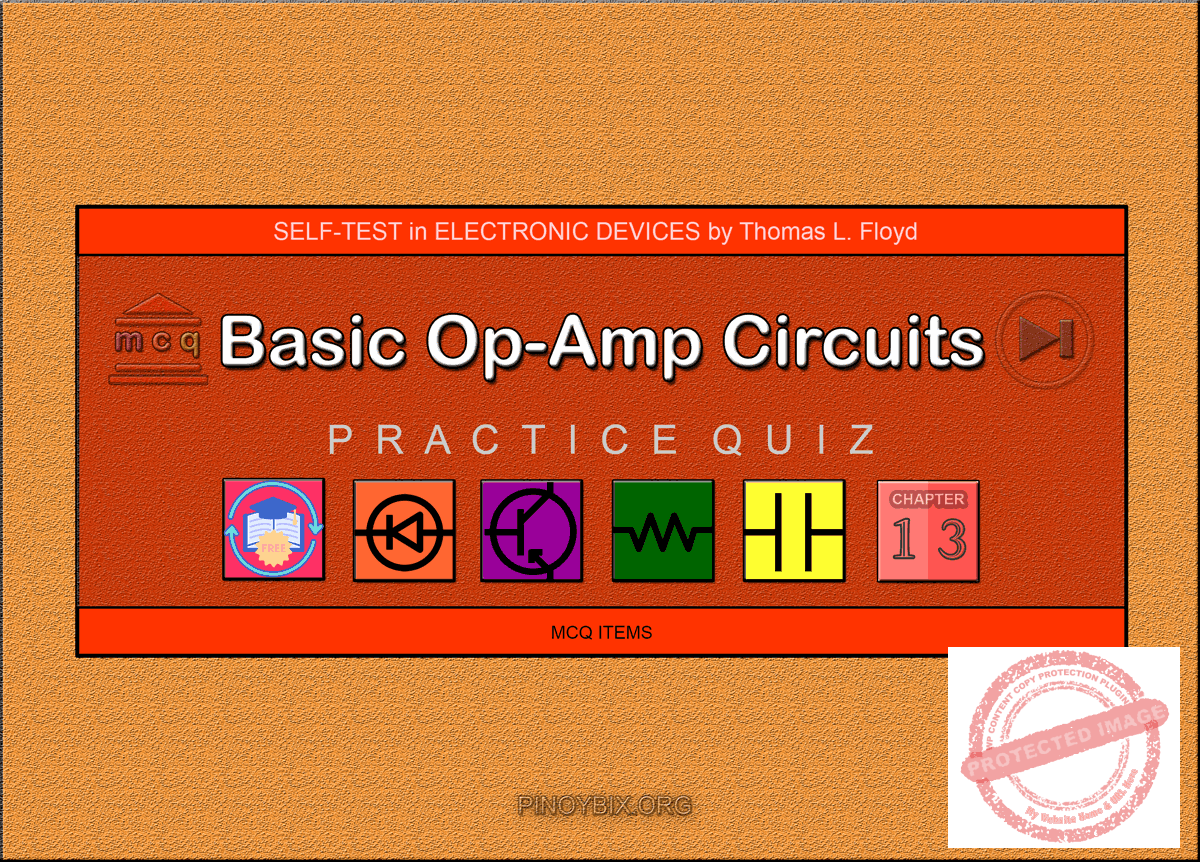# Floyd Self-test in Basic Op-Amp Circuits

(Last Updated On: December 7, 2019)This is the Self-test in Chapter 13: Basic Op-Amp Circuits from the book Electronic Devices Conventional Current Version, 9th edition by Thomas L. Floyd. If you are looking for a reviewer in Electronics Engineering this will definitely help you before taking the Board Exam.

#### Floyd Self-test Chapter 13 Topic Outline

• Floyd Self-test in Comparators
• Floyd Self-test in Summing Amplifiers
• Floyd Self-test in Integrators and Differentiators

If you are looking for the Multiple Choice Questions in Floyd’s Electronic Devices proceed to

#### Start Practice Exam Test Questions

Choose the letter of the best answer in each questions.

1. In a zero-level detector, the output changes state when the input

(a) is positive

(b) is negative

(c) crosses zero

(d) has a zero rate of change

Solution:

2. The zero-level detector is one application of a

(a) comparator

(b) differentiator

(c) summing amplifier

(d) diode

Solution:

3. Noise on the input of a comparator can cause the output to

(a) hang up in one state

(b) go to zero

(c) change back and forth erratically between two states

(d) produce the amplified noise signal

Solution:

4. The effects of noise can be reduced by

(a) lowering the supply voltage

(b) using positive feedback

(c) using negative feedback

(d) using hysteresis

Solution:

5. A comparator with hysteresis

(a) has one trigger point

(b) has two trigger points

(c) has a variable trigger point

(d) is like a magnetic circuit

Solution:

6. In a comparator with hysteresis,

(a) a bias voltage is applied between the two inputs

(b) only one supply voltage is used

(c) a portion of the output is fed back to the inverting input

(d) a portion of the output is fed back to the noninverting input

Solution:

7. Using output bounding in a comparator

(a) makes it faster

(b) keeps the output positive

(c) limits the output levels

(d) stabilizes the output

Solution:

8. A summing amplifier can have

(a) only one input

(b) only two inputs

(c) any number of inputs

Solution:

9. If the voltage gain for each input of a summing amplifier with a feedback resistor is unity, the input resistors must have a value of

(a) 4.7kΩ

(b) 4.7kΩ divided by the number of inputs

(c) 4.7kΩ times the number of inputs

Solution:

10. An averaging amplifier has five inputs. The ratio Rf /R must be

(a) 5

(b) 0.2

(c) 1

Solution:

11. In a scaling adder, the input resistors are

(a) all the same value

(b) all of different values

(c) each proportional to the weight of its input

(d) related by a factor of two

Solution:

12. In an ideal integrator, the feedback element is a

(a) resistor

(b) capacitor

(c) zener diode

(d) voltage divider

Solution:

13. For a step input, the output of an integrator is

(a) a pulse

(b) a triangular waveform

(c) a spike

(d) a ramp

Solution:

14. The rate of change of an integrator’s output voltage in response to a step input is set by

(a) the RC time constant

(b) the amplitude of the step input

(c) the current through the capacitor

(d) all of these

Solution:

15. In a differentiator, the feedback element is a

(a) resistor

(b) capacitor

(c) zener diode

(d) voltage divider

Solution:

16. The output of a differentiator is proportional to

(a) the RC time constant

(b) the rate at which the input is changing

(c) the amplitude of the input

Solution:

17. When you apply a triangular waveform to the input of a differentiator, the output is

(a) a dc level

(b) an inverted triangular waveform

(c) a square waveform

(d) the first harmonic of the triangular waveform

Solution:

### Complete List of Floyd Self-test in Electronic Devices

Show Simple Act of Caring!

Please consider subscribing to our YouTube Channel for engineering video tutorials. You can also find us on Twitter and Facebook. Lastly, Our app is now available on Google Play, Pinoybix Elex

© 2014 PinoyBIX Engineering. © 2019 All Rights Reserved | How to Donate? |#### Pre-board in Electronics Engineering

Pre-board in Electronics Engineering - Answers

#### Pre-board in Communications Engineering

Pre-board in Communications Engineering - Answers

#### GEAS Solution

Dynamics problem Economics problem Physics problem Statics problem Strength problem Thermodynamics problem

#### Questions and Answers in GEAS

Engineering Economics Engineering Laws and Ethics Engineering Management Engineering Materials Engineering Mechanics General Chemistry Physics Strength of Materials Thermodynamics
Consider Simple Act of Caring!: LIKE MY FB PAGE

Our app is now available on Google Play, Pinoybix Elex